# Solutions, mixtures

How many liters of 70% solution we must add to 5 liters of 30% solution to give us a 60% solution?

Result

x =  15 l

#### Solution:

x * 0.70 + 5* 0.30 = 0.60 * (x+5)

0.1x = 1.5

x = 15

Calculated by our simple equation calculator.

Leave us a comment of example and its solution (i.e. if it is still somewhat unclear...):

Showing 0 comments:Be the first to comment!#### To solve this example are needed these knowledge from mathematics:

Our percentage calculator will help you quickly calculate various typical tasks with percentages. Do you have a linear equation or system of equations and looking for its solution? Or do you have quadratic equation? Tip: Our volume units converter will help you with converion of volume units.

## Next similar examples:

1. Concentration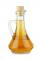How 0.5 liters of 8% vinegar diluted to a concentration of 20 hundredths % of vinegar? How many liters of water must be pour?
2. Alcohol mixtureThree liters of 96 percent alcohol we set up a certain amount of distilled water to form a 54-percent alcohol. How many liters of distilled water were used?
3. Assistant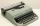Assistant rewrote 15% of the entire manuscript in 12 hours. How many hours must still write to rewrite the rest of the manuscript?
4. Highway repairThe highway repair was planned for 15 days. However, it was reduced by 30%. How many days did the repair of the highway last?
5. PercentsHow many percents is 900 greater than the number 750?
6. Cows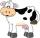Agricultural cooperative has increased the number of housed cows by 14% to 285 units. By how many cows increased agricultural cooperative the number of cows?
7. Sale discountThe product was discounted so that eight products at a new price cost just as five products at an old price. How many percents is the new price lower than the old price?
8. Sales offThe price has decreased by 20%. How many percents do I have to raise the new price to be the same as before the cut?
9. Barrel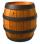The barrel is filled with water up to 57% of its capacity. When we add two buckets will be filled to 73% of its capacity. What is the volume of a barrel, if the volume of the bucket is 12 liters?
10. Percentage - fractionsAbout what percentage we must increase number 1/6 to get number 1/3?
11. Finding the baseFind unknown base of percent: 12.5 percent of what = 16 ?
12. Sale offProduct cost 95 euros before sale off. After sale off cost 74 euros and 10 cents. About what percentage of produt became cheaper?
13. Ethnicity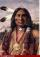Share of ethnicity XY is 26%, which is 1/9 more than in the prewar period. What was the share of that ethnicity in the pre-war period?
14. Cargo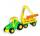Car with cargo weight 7610 kg. Weight of car is 23% of the total weight of car and cargo. How heavy is cargo?
15. The priceThe price of the land increased by 17%. What was the original price of the land if it now costs 46800 €?
16. Goods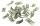To the market is introducing a new product, the first week is sold at a reduced price. 8 products is sell at an entry price same as 5 products at the normal price. How much % is reduced price less than the current price for this product?
17. Reducing numberReducing the an unknown number by 28.5% we get number 243.1. Determine unknown number.